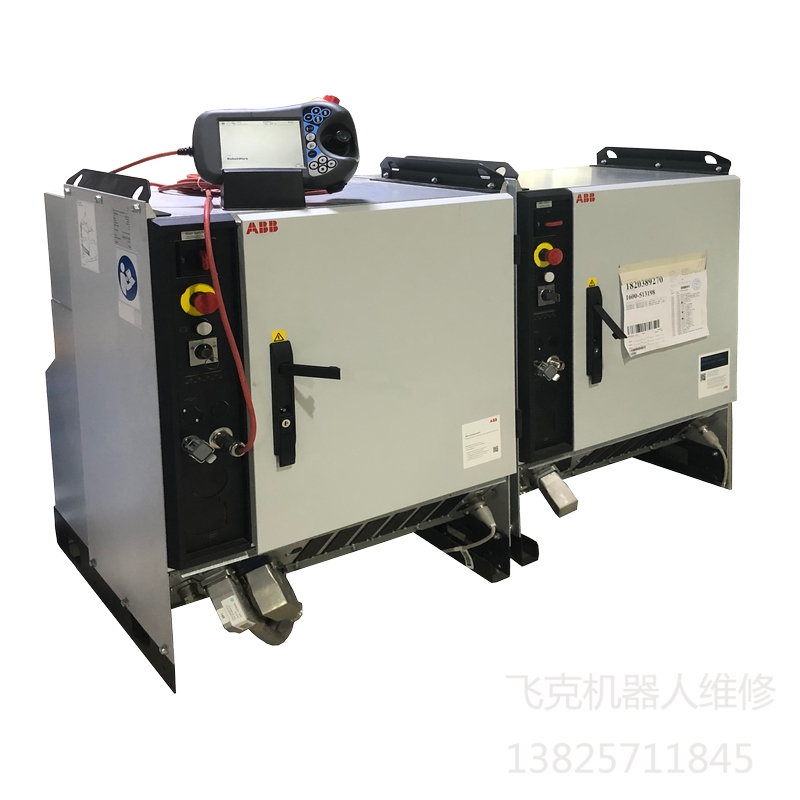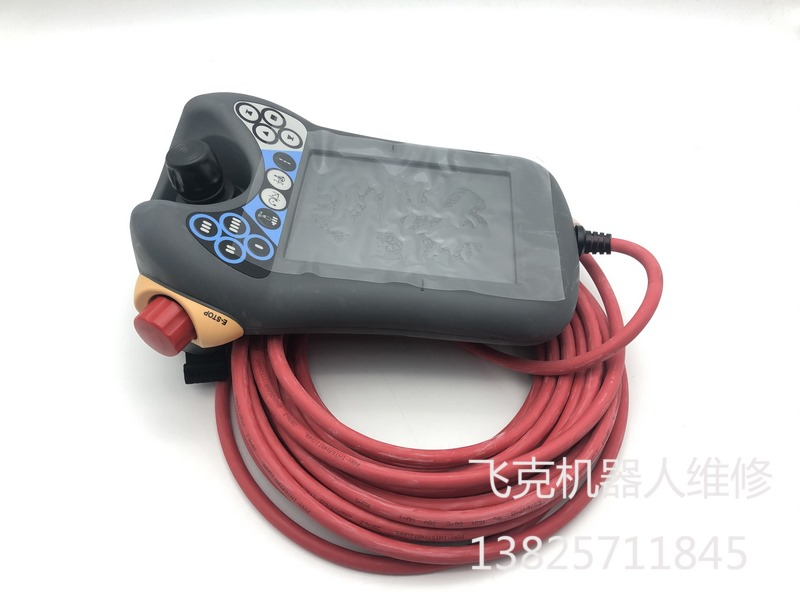当前位置：工业机器人维修 >> ABB维修案例

ABB维修案例

# ABB机器人日常维护之指令开发示例

RAPID应用被称作一项任务。一项任务包括一组模块。一个模块包含一组数据和程序声明。

Atomic(内置原子型/基本数据类型)数据类型：

bool为枚举类型，其值为真或假，提供了一种开展逻辑计算和关联计算的方式。

num型支持精确算术计算和近似算术计算。

string型表示字符序列。Record（记录型）数据类型：为一种带命名有序分量的复合类型。record类型的值为由各分量的值组成的复合值。一个分量可具备atomic型或record型，用户自定义复杂数据类型一般就是记录型。内置记录型有：

pos型表示空间位置（矢量）。

orient型表示在空间中的方位。

pose型表示坐标系（位置/方位组合）。alias数据类型被定义为等同于另一种类型。alias类型提供一种对象分类手段。系统可采用alias分类来查找和显示与类型相关的对象，内置别名型数据有errnum和intnum。Errnum和intnum均为num的别名，用于表示错误和中断编号。

Rapid程序分为三类：无返回值程序（PROC）、有返回值程序（FUNC）和软中断程序。程序声明指定了程序的下列内容：名称，数据类型（仅对有返回值程序有效），参数（不适用于软中断程序），数据声明和语句（体），回退处理器（仅对无返回值程序有效），错误处理器和撤销处理器ABB机器人控制柜维修,ABB机器人示教器维修,ABB机器人I/O板维修,ABB机器人驱动器维修,ABB机器人伺服电机维修,ABB机器人计算机板维修,ABB机器人电源板维修,ABB机器人安全板维修

FUNC robtarget pStack(robtarget pBase,num partColumns,num partWidth,num PartHeight,num numCounter)
VAR num Columns;
VAR num Rows;
Columns:=( numCounter -1) MOD partColumns;
Rows:=( numCounter -1-Columns)/partColumns;
RETURN Offs(pBase,0,Columns*(PartWidth),Rows*PartHeight);
ENDFUNC

FUNC robtarget getRobT(switch Rel,num x,num y,num z,inout tooldata tool,wobjdata wobj)
VAR robtarget pCurrent;
pCurrent:=crobT(tool:=toolwobj:=wobj);
IF Present(Rel) THEN
IF Present(x) THEN
pCurrent.trans.x:=pCurrent.trans.x+x;
ENDIF
IF Present(y) THEN
pCurrent.trans.y:=pCurrent.trans.y+y;
ENDIF
IF Present(z) THEN
pCurrent.trans.z:=pCurrent.trans.z+z;
ENDIF
ELSE
IF Present(x) THEN
pCurrent.trans.x:=x;
ENDIF
IF Present(y) THEN
pCurrent.trans.y:=y;
ENDIF
IF Present(z) THEN
pCurrent.trans.z:=z;
ENDIF
ENDIF
RETURN pCurrent;
tENDFUNC

movel getRobPos(rel,y:=100, ,tool0,wobj0),v100,z0,tool0;
movel getRobPos(z:=1000,tool0,wobj0),v100,z0,tool0;
p1:= getRobPos(y:=200,tool0,wobj0),

FUNC bool rCheckPos(jointtarget pCheck,num num1)
VAR jointtarget pCurrent;
VAR num numTemp;
IF Present(num1) THEN
numTemp:=num1;
ENDIF
pCurrent:=CalcJointT(CRobT(),tool0);
IF pCurrent.robax.rax_1<=pcheck.robax.rax_1+num1 and="" pcurrent.robax.rax_1="">=pCheck.robax.rax_1-num1 AND
pCurrent.robax.rax_2<=pcheck.robax.rax_2+num1 and="" pcurrent.robax.rax_2="">=pCheck.robax.rax_2-num1 AND
pCurrent.robax.rax_3<=pcheck.robax.rax_3+num1 and="" pcurrent.robax.rax_3="">=pCheck.robax.rax_3-num1 AND
pCurrent.robax.rax_4<=pcheck.robax.rax_4+num1 and="" pcurrent.robax.rax_4="">=pCheck.robax.rax_4-num1 AND
pCurrent.robax.rax_5<=pcheck.robax.rax_5+num1 and="" pcurrent.robax.rax_5="">=pCheck.robax.rax_5-num1 AND
pCurrent.robax.rax_6<=pcheck.robax.rax_6+num1 and="" pcurrent.robax.rax_6="">=pCheck.robax.rax_6-num1 THEN
RETURN TRUE;
ELSE
RETURN FALSE;
ENDIF
ENDFUNC